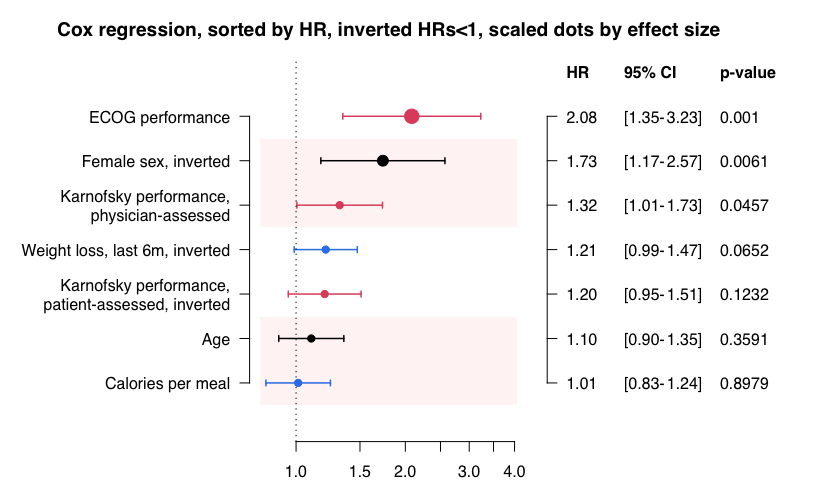forplo - flexible forest plots

Background

forplo is an R package meant to simplify the creation and customization of forest plots (alternatively called dot-and-whisker plots). Input classes accepted by forplo are data.frame, matrix, lm, glm, and coxph. forplo was written in base R and does not depend on other packages.

Examples: data.frames

With forplo, a data.frame or matrix with exactly 3 columns can be entered in the mat argument, where the first column will be interpreted as the effect estimate and the second and third columns are interpreted as the lower and upper bounds of a confidence interval. By default, the row names of mat will be used as variable names. Alternatively, one can specify the variable names directly by entering a character vector of the same length as the number of rows in mat under row.labels. The left margin of the plot is automatically resized to fit the variable names, but it can be handset by changing margin.left. This goes for the other margins as well.

The following examples will illustrate the use of forplo and how the arguments may be used for customization. The data for the first example are due to Joanne E. McKenzie and Sue E. Brennan, chapter 12 of the Cochrane Handbook for Systematic Reviews of Interventions, version 6.2 (2021).

exdf <- cbind(OR=c(1.21,0.90,1.02,
1.54,1.32,0.79,1.38,0.85,1.11,
1.58,1.80,2.27),
LCI=c(0.82,0.61,0.66,
1.08,0.91,0.48,1.15,0.39,0.91,
0.99,1.48,0.92),
UCI=c(1.79,1.34,1.57,
2.19,1.92,1.32,1.64,1.87,1.34,
2.54,2.19,5.59),
groups=c(1,1,1,
2,2,2,2,2,2,
3,3,3))
exdf <- data.frame(exdf)
rownames(exdf) <- c('Barry, 2005', 'Frances, 2000', 'Rowley, 1995',
'Biro, 2000', 'Crowe, 2010', 'Harvey, 1996',
'Johns, 2004', 'Parr, 2002', 'Zhang, 2011',
'Flint, 1989', 'Mac Vicar, 1993', 'Turnbull, 1996')
knitr::kable(exdf)
OR LCI UCI groups
Barry, 2005 1.21 0.82 1.79 1
Frances, 2000 0.90 0.61 1.34 1
Rowley, 1995 1.02 0.66 1.57 1
Biro, 2000 1.54 1.08 2.19 2
Crowe, 2010 1.32 0.91 1.92 2
Harvey, 1996 0.79 0.48 1.32 2
Johns, 2004 1.38 1.15 1.64 2
Parr, 2002 0.85 0.39 1.87 2
Zhang, 2011 1.11 0.91 1.34 2
Flint, 1989 1.58 0.99 2.54 3
Mac Vicar, 1993 1.80 1.48 2.19 3
Turnbull, 1996 2.27 0.92 5.59 3
forplo(exdf[,1:3])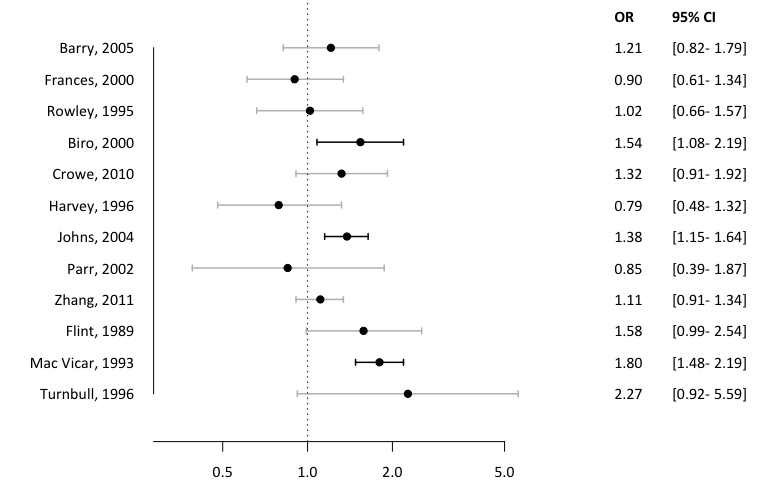This is the default output when entering a data.frame. Now we will group the data by a grouping variable. When using groups, the argument groups is used to specify the group membership of each variable in a numeric vector (if is not numeric, it will be coerced to numeric), and a character vector of group labels of length(unique(groups)) should be entered as grouplabs. If the variables are not in order of group they will be automatically sorted by group before being plotted.

forplo(exdf[,1:3],
groups=exdfgroups, grouplabs=c('Low risk of bias', 'Some concerns', 'High risk of bias'))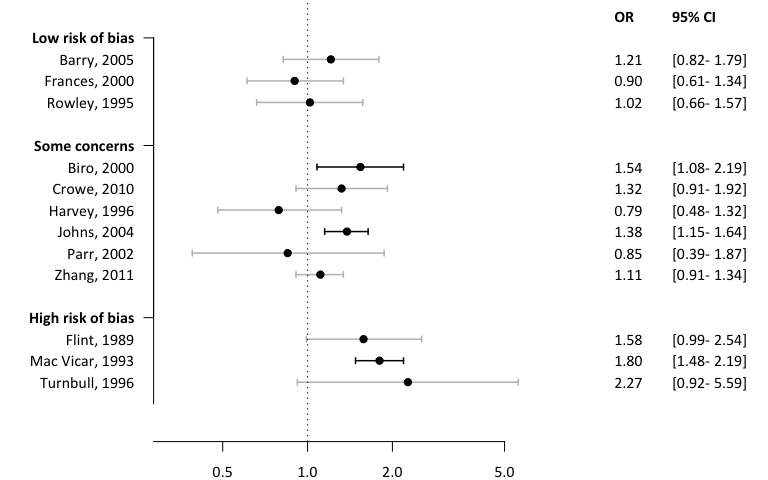To demonstrate some more of the features of forplo, we will meta-analyze the studies in each group and across all studies, and create a new data.frame containing the meta-analytic estimates. We can add more columns (log OR, SE, weights) to the plot using add.columns and add.colnames. We can use diamonds to indicate meta-analytic estimates. In order to do this, we specify which estimates are diamonds by giving a numeric vector to diamond. Specific customization options for the diamonds are diamond.col and diamond.line (TRUE or FALSE). The diamond line is only shown for the last diamond, on the assumption that this would be the overall meta-estimate. To make the plot a bit more interesting, we will also add some color with col and remove the edges from the confidence intervals by setting ci.edge=FALSE. We will also left align the group and study names with left.align==TRUE. logORs <- round(log(exdfOR),2)
SE <- round((log(exdf$UCI)-logORs)/1.96,2) meta1 <- meta::metagen(logORs[1:3],SE[1:3]) meta2 <- meta::metagen(logORs[4:9],SE[4:9]) meta3 <- meta::metagen(logORs[10:12],SE[10:12]) metatot <- meta::metagen(logORs,SE) # create new data.frame exdf2 <- exdf exdf2$logORs <- logORs
exdf2$SE <- SE exdf2$weights <- round(metatot$w.random/sum(metatot$w.random)*100,2)
exdf2 <- rbind(subset(exdf2,groups==1),
c(round(exp(meta1$TE.random),2), round(exp(meta1$lower.random),2),
round(exp(meta1$upper.random),2), 1,round(meta1$TE.random,2),round(meta1$seTE.random,2),sum(exdf2$weights[1:3])),
subset(exdf2,groups==2),
c(round(exp(meta2$TE.random),2), round(exp(meta2$lower.random),2),
round(exp(meta2$upper.random),2), 2,round(meta2$TE.random,2),round(meta2$seTE.random,2),sum(exdf2$weights[4:9])),
subset(exdf2,groups==3),
c(round(exp(meta3$TE.random),2), round(exp(meta3$lower.random),2),
round(exp(meta3$upper.random),2), 3,round(meta3$TE.random,2),round(meta3$seTE.random,2),sum(exdf2$weights[10:12])),
c(round(exp(metatot$TE.random),2), round(exp(metatot$lower.random),2),
round(exp(metatot$upper.random),2), 4,round(metatot$TE.random,2),round(metatot$seTE.random,2),100)) exdf2 <- data.frame(exdf2) rownames(exdf2)[c(4,11,15,16)] <- c('Diamond 1','Diamond 2','Diamond 3','Diamond 4') # show new data.frame knitr::kable(exdf2) OR LCI UCI groups logORs SE weights Barry, 2005 1.21 0.82 1.79 1 0.19 0.20 8.14 Frances, 2000 0.90 0.61 1.34 1 -0.11 0.21 7.73 Rowley, 1995 1.02 0.66 1.57 1 0.02 0.22 7.33 Diamond 1 1.04 0.82 1.32 1 0.04 0.12 23.20 Biro, 2000 1.54 1.08 2.19 2 0.43 0.18 9.05 Crowe, 2010 1.32 0.91 1.92 2 0.28 0.19 8.58 Harvey, 1996 0.79 0.48 1.32 2 -0.24 0.26 5.97 Johns, 2004 1.38 1.15 1.64 2 0.32 0.09 14.03 Parr, 2002 0.85 0.39 1.87 2 -0.16 0.40 3.16 Zhang, 2011 1.11 0.91 1.34 2 0.10 0.10 13.45 Diamond 2 1.22 1.04 1.44 2 0.20 0.08 54.24 Flint, 1989 1.58 0.99 2.54 3 0.46 0.24 6.61 Mac Vicar, 1993 1.80 1.48 2.19 3 0.59 0.10 13.45 Turnbull, 1996 2.27 0.92 5.59 3 0.82 0.46 2.50 Diamond 3 1.79 1.50 2.13 3 0.58 0.09 22.56 Diamond 4 1.27 1.09 1.48 4 0.24 0.08 100.00 forplo(exdf2[,1:3], groups=exdf2$groups,
grouplabs=c('Low risk of bias',
'Some concerns',
'High risk of bias',
'Overall'),
left.align=TRUE,
col=2,
ci.edge=FALSE,
diamond=c(4,11,15,16),
diamond.col='#b51b35')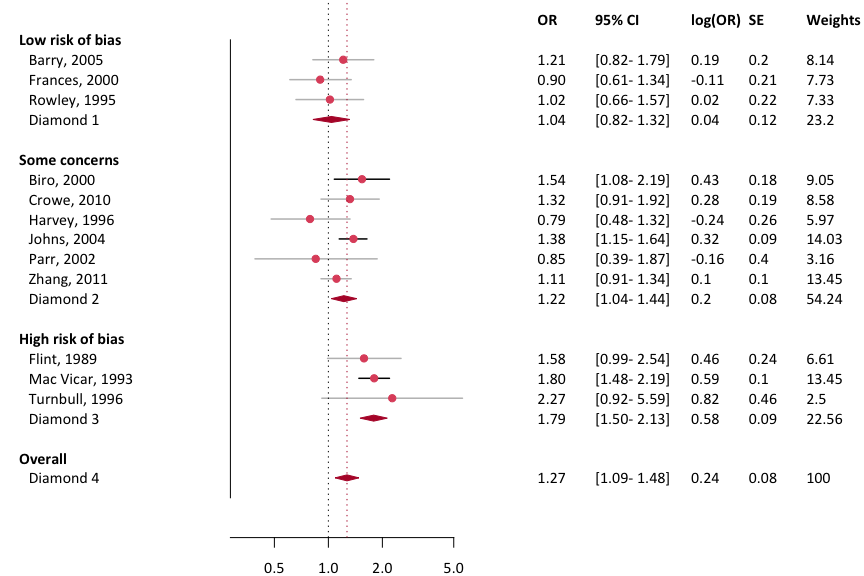Let's try some more customization options. First, we will make the size of the dots proportional to the study weights with scaledot.by (a numeric vector, for example containing sample sizes or weights). We will remove the diamond weights to get better relative scaling here (in scaledot.by, the maximum value is taken as the reference value). We will also change the character type with char, and add text underneath the x-axis with favorlabs. Finally, we will shade every other row using the shade.every argument. This latter argument can take on any value, to allow visual grouping by line color.

weights <- exdf2$weights weights[c(4,11,15,16)] <- NA forplo(exdf2[,1:3], groups=exdf2$groups,
grouplabs=c('Low risk of bias',
'Some concerns',
'High risk of bias',
'Overall'),
left.align=TRUE,
add.columns=exdf2$weights, add.colnames=c('Weights'), col=2, char=15, ci.edge=FALSE, diamond=c(4,11,15,16), diamond.col='#b51b35', scaledot.by=weights, favorlabs=c('Favours other models','Favours midwife-led'), shade.every=1)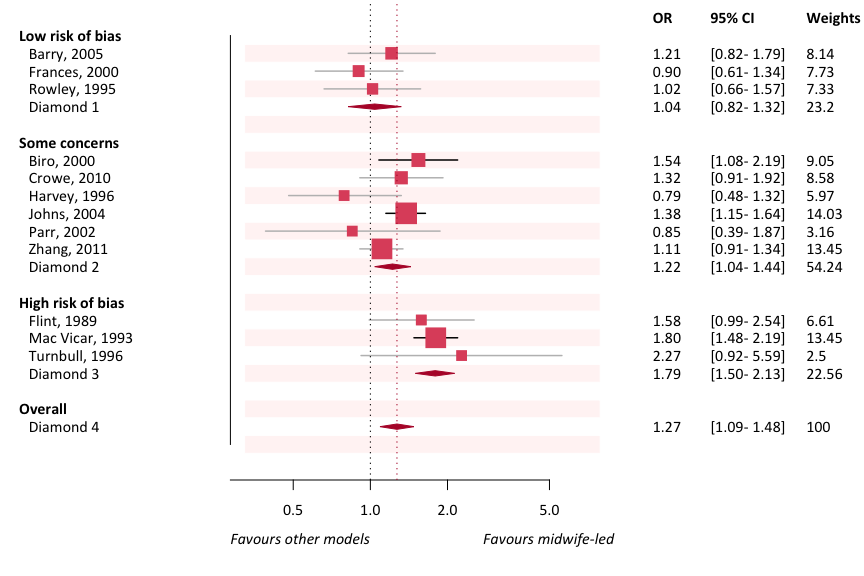Other possibilities include the option to sort rows by effect size, to invert odds ratios and confidence intervals using flipbelow1=TRUE or by specifying the relevant rows with fliprow, adding ticks to the left bar or right bar (leftbar.ticks and rightbar.ticks, which require left.bar and right.bar to be TRUE), and to increase (or decrease) the empty space between groups by changing group.space. Further, the dots and CIs can also be colored by a grouping variable, using the arguments fill.by for the grouping variable and fill.colors to designate the corresponding colors. The font can be set with font. Note: monospace fonts don't work well with the automatic setting of plot margins. forplo(exdf2[,1:3], groups=exdf2$groups,
grouplabs=c('Low risk of bias',
'Some concerns',
'High risk of bias',
'Overall'),
left.align=TRUE,
add.columns=exdf2$weights, add.colnames=c('Weights'), ci.edge=FALSE, diamond=c(4,11,15,16), diamond.col=adjustcolor(4,0.8), scaledot.by=weights, favorlabs=c('Favours other models','Favours midwife-led'), shade.every=1, shade.col='gray', font='Garamond', fill.by=exdf2$groups,
fill.colors=c('#f54251','#1403fc','#fc03a5',1),
title='Example of a plot with custom colors and font',
margin.left=9)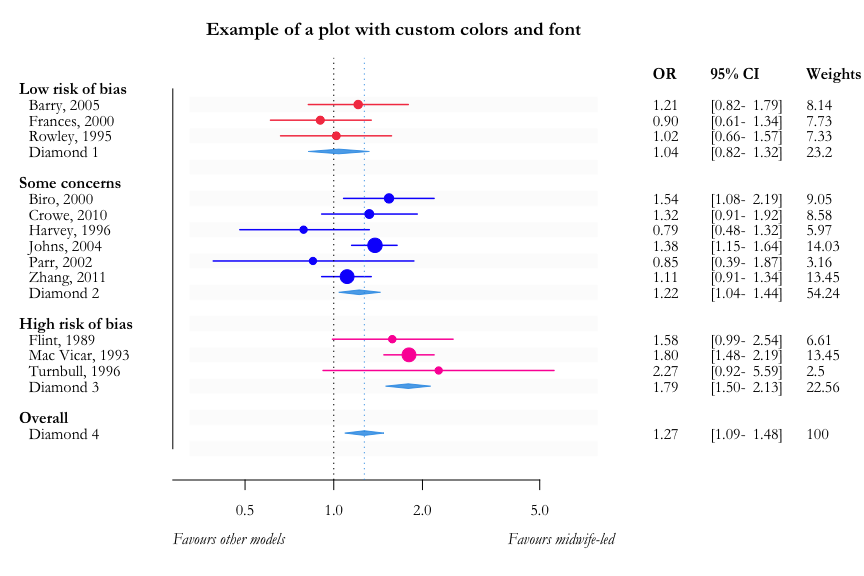Saving plots with forplo

forplo can save plots as one of two types, .png or .wmf. For this, the argument save should be set to TRUE, the desired folder should be specified in save.path, the name of the plot in save.name, and the file type should be designated with save.type (default is .png). The width and height of the plot (in inches) can be set with save.width and save.height.

More examples: regression models

forplo also accepts models of class lm, glm and coxph to enable fast visualization of commonly used regression models. P-values given by summary() are shown, and profiling confidence intervals computed. The corresponding effect measure (e.g. odds ratios for logistic regression models) is automatically shown. All customization options are still available when using models as input.

First let's define the models.

mod1 <- lm(Sepal.Length~Sepal.Width+Species+Petal.Width+Petal.Length,iris)
mod2 <- glm(as.numeric(status==2)~scale(age)+sex+
ph.ecog+scale(ph.karno)+scale(pat.karno)+
scale(meal.cal)+scale(wt.loss),survival::lung,family=binomial)
survmod <- survival::coxph(survival::Surv(time,status)~scale(age)+sex+
ph.ecog+scale(ph.karno)+scale(pat.karno)+
scale(meal.cal)+scale(wt.loss),survival::lung)

This is the default plot when entering a linear regression model.

forplo(mod1)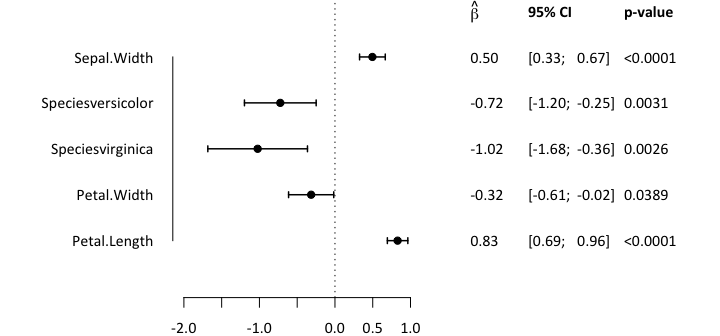The following are some examples of customized plots using lm, glm and coxph models as input.

forplo(mod1,
row.labels=c('Sepal width','Versicolor','Virginica','Petal width','Petal length'),
groups=c(1,2,2,3,3),
grouplabs=c('Sepal traits','Species','Petal traits'),
left.align=TRUE,
xlim=c(-2,2),
title='Linear regression with grouped estimates')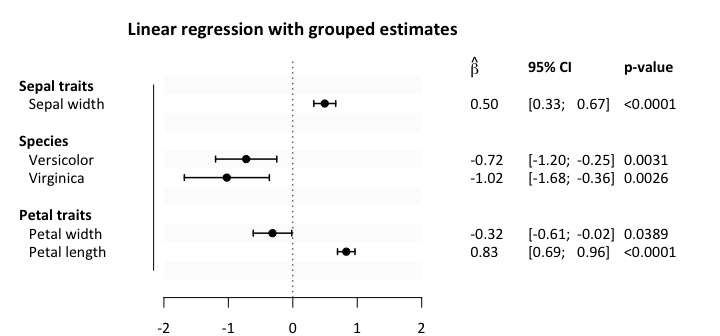The plot window may have to be resized to show all labels optimally.

forplo(mod2,
sort=TRUE,
favorlabs=c('Lower mortality','Higher mortality'),
ci.edge=FALSE,
char=18,
row.labels=c('Age',
'Female sex',
'ECOG performance',
'Karnofsky performance,\nphysician-assessed',
'Karnofsky performance,\npatient-assessed',
'Calories per meal',
'Weight loss, last 6m'),
title='Logistic regression, sorted by OR')
#> Waiting for profiling to be done...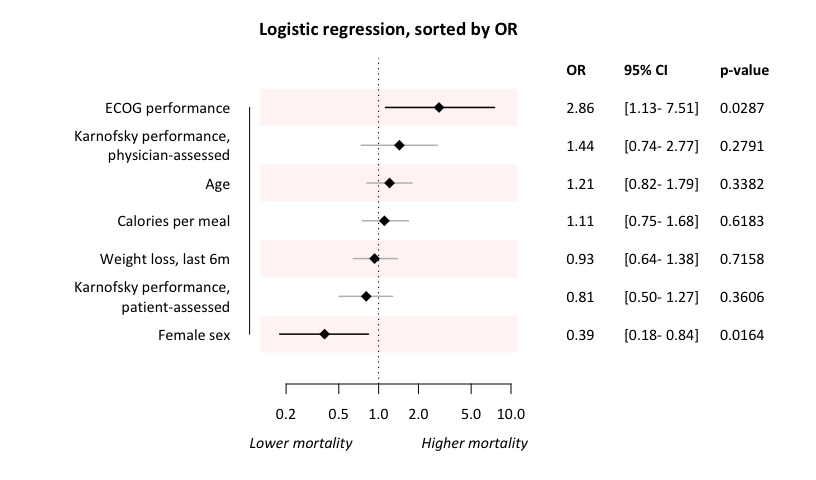forplo(survmod,
row.labels=c('Age',
'Female sex',
'ECOG performance',
'Karnofsky performance,\nphysician-assessed',
'Karnofsky performance,\npatient-assessed',
'Calories per meal',
'Weight loss, last 6m'),
sort=TRUE,
flipbelow1=TRUE,
flipsymbol=', inverted',
fill.by=c(1,1,2,2,2,3,3),
fill.colors=c(1,2,'#3483eb'),
scaledot.by=abs(coef(survmod)),